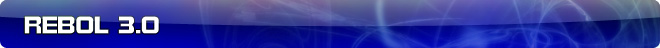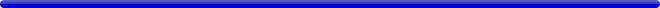Carl Sassenrath, CTO REBOL Technologies 3-Apr-2007 3:04 GMT Article #0079

The main purpose of the vector datatype is to provide more efficient integer and decimal array storage.

For example, if you need to index a large file and keep track of 100000 index positions, you can do so with the block:

```idxs: make block! 100000
```

or, you can use:

```idxs: make vector! 100000
```

The benefit of the vector is the memory it saves. Of course, the downside is that all its elements must be of the same type and size (homogeneous, e.g. 32 bit integers). In the example above, the vector array would save 1'200'000 bytes of memory. So, that is a win in many such cases.

The above make creates default elements as 32 bit signed integers. However, you can specify the bit-size of the vector element. For example, if you need to store a sound that consisted of 100000 samples at 16 bits-per-sample:

```samp: make vector! [integer! 16 100000]
```

If you needed those to be unsigned integers:

```samp: make vector! [unsigned integer! 16 100000]
```

Note that only 8, 16, 32, and 64 bit sizes are supported.

Instead of integers, if you want to store decimal (floating point) numbers:

```decs: make vector! [decimal! 32 100000]
```

or:

```decs: make vector! [decimal! 64 100000]
```

Note that decimals must be 32 or 64 bits and are always signed numbers.

### Element Access

Currently, the only access to vector elements is via absolute indices. The series index methods (first, next, etc.) are not supported at this time.

To get a value:

```v: pick vect 100
```

or,

```v: vect/100
```

To set a value:

```poke vect 100 10
```

or

```vect/100: 10
```

The integer value for elements makes vectors more appropriate for mathematical functions such as those that deal with sound samples. For example, to generate a 16 bit sine wave sample:

```wave: make vector! [integer! 16 3600]
repeat n 3600 [
poke wave n 32767 * sine n / 10
]
```

We can support other native actions, as we find them to be useful. For example, we may want to provide a high-speed method of setting a sequence of values.

### Initializers and Conversions

You can initialize a vector from a block using code such as:

```vect: make vector! [integer! 32 [1 20 300 40 -50 ...]]
```

In addition, a direct conversion method will be supported:

```vect: to vector! [1 20 300 40 -50 ...]
```

In such cases the type and size will be inferred from the data itself to create the most compact vector representation.

Conversions from vectors to blocks and binaries will also be supported.

```blk: to block! vect
bin: to binary! vect
```

Details to be defined.

### Dimensions

It is planned for vectors to support multiple dimensions. To define a two dimensional vector of 100 vectors of 365 integers.

```samp: make vector! [integer! 32 100 365]

print samp/30/10
samp/50/200: 100
```

This is not yet supported, so let me know if you need it.

### Bounds and Range Checks

Currently, index bounds are checked, but element ranges are not. For example, no error occurs if a 16 bit integer is set to 32 bits. The result is truncated. It is possible to add element range checks at the cost of additional performance overhead and code size.

### Vector Operators

Vector operators like add, multiply, inverse, determinant, etc, are not planned for the first release of REBOL 3. They can be added later, if so desired.

### Other Datatypes

In the future, it may be possible to allow other datatypes to be vectorized.